# T-ideal

(diff) ← Older revision | Latest revision (diff) | Newer revision → (diff)

of a free associative algebra

A totally invariant ideal, that is, an ideal invariant under all endomorphisms. The set of all polynomial identities of an arbitrary variety of associative algebras over a field(cf. Associative rings and algebras) forms a-ideal in the countably-generated free algebra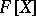,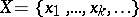. Thus, there exists a one-to-one correspondence between the-ideals ofand the varieties of associative algebras over. Ifhas characteristic, then for every-idealthere exists a natural number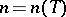such that certain powers of elements ofare elements of, and only they, where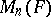is the ideal of identities of the algebra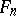of all-matrices over. In this case a-ideal can also be defined as a (two-sided) ideal that is closed under all differentiations of the free algebra. The quotient algebrais a PI-algebra withas set of polynomial identities. It is called the relatively free algebra (or generic algebra) with-ideal of identities(and is a free algebra in the variety of algebras defined by the identities in). The algebrahas no zero divisors if and only iffor some natural number. Every-idealof a free associative algebra is primary.

The-ideals of a free associative algebra on infinitely many generators over a field of characteristic zero form a free semi-group under the operation of multiplication of ideals. In this case a-ideal can be defined as an ideal invariant under all automorphisms of the free algebra.

For the question as to whether every-ideal ofis the totally invariant closure of finitely many elements (Specht's problem) see also Variety of rings.-ideals can be defined for non-associative algebras (Lie, alternative and others) by analogy with the associative case.

How to Cite This Entry:
T-ideal. Encyclopedia of Mathematics. URL: http://encyclopediaofmath.org/index.php?title=T-ideal&oldid=14127
This article was adapted from an original article by V.N. Latyshev (originator), which appeared in Encyclopedia of Mathematics - ISBN 1402006098. See original article